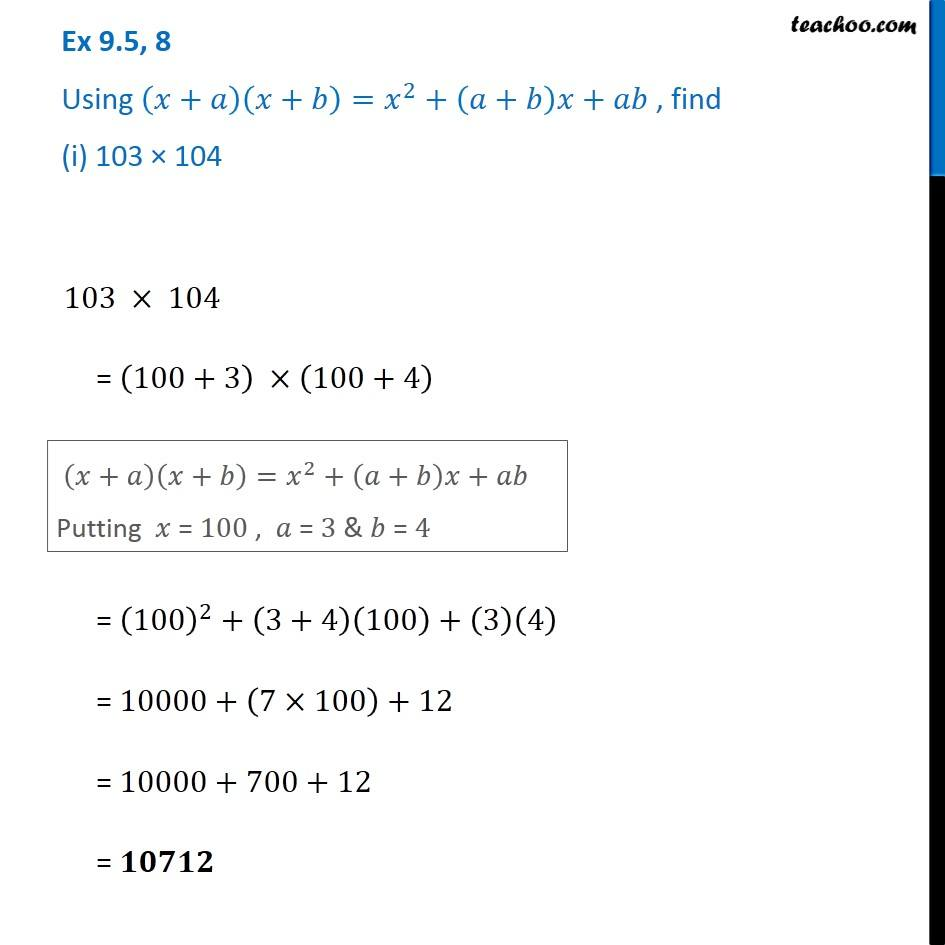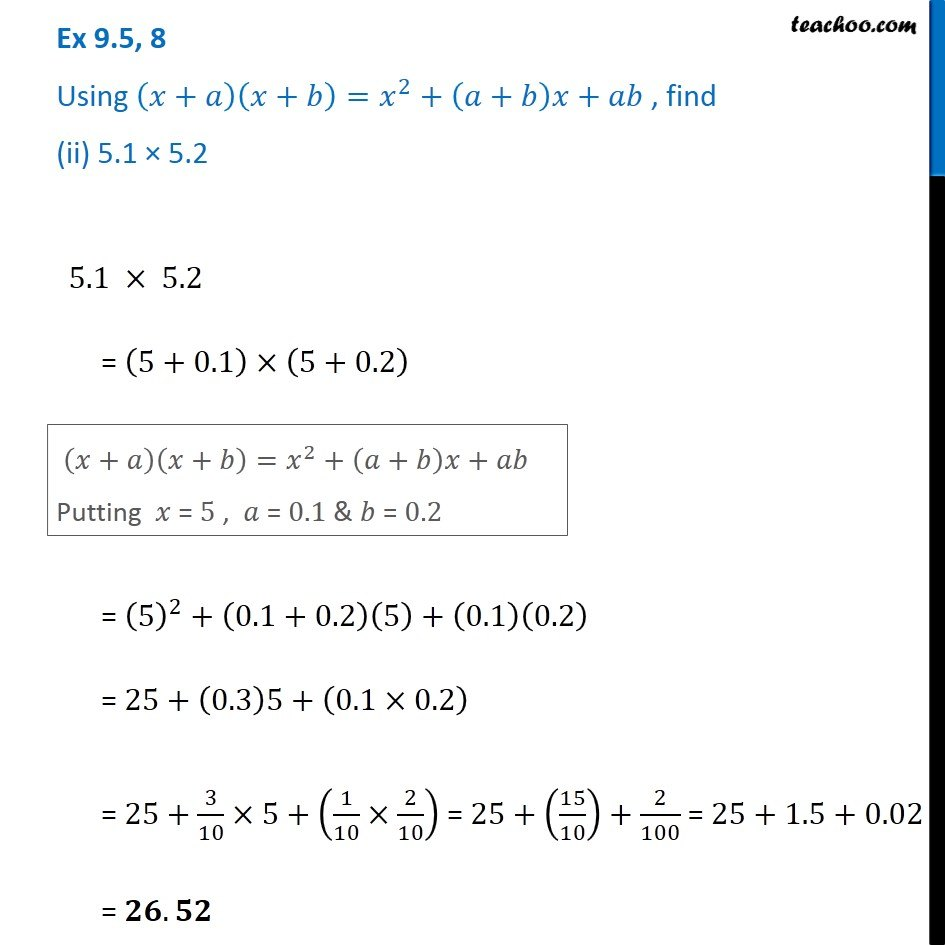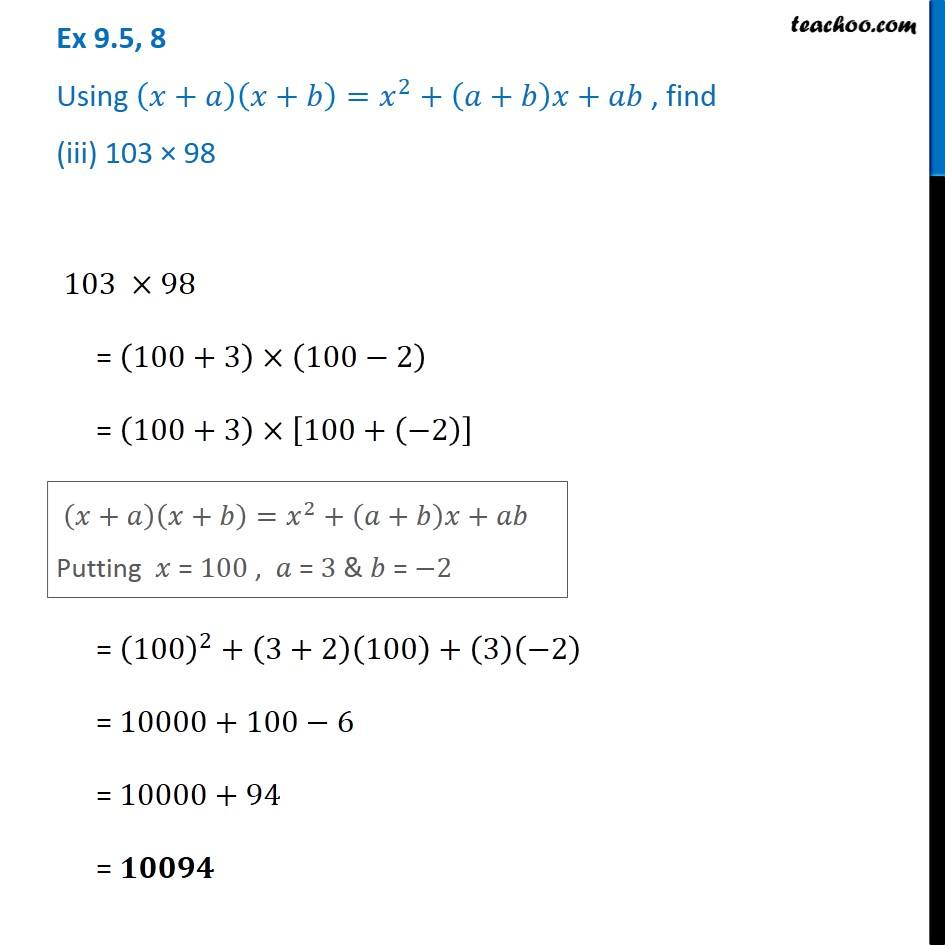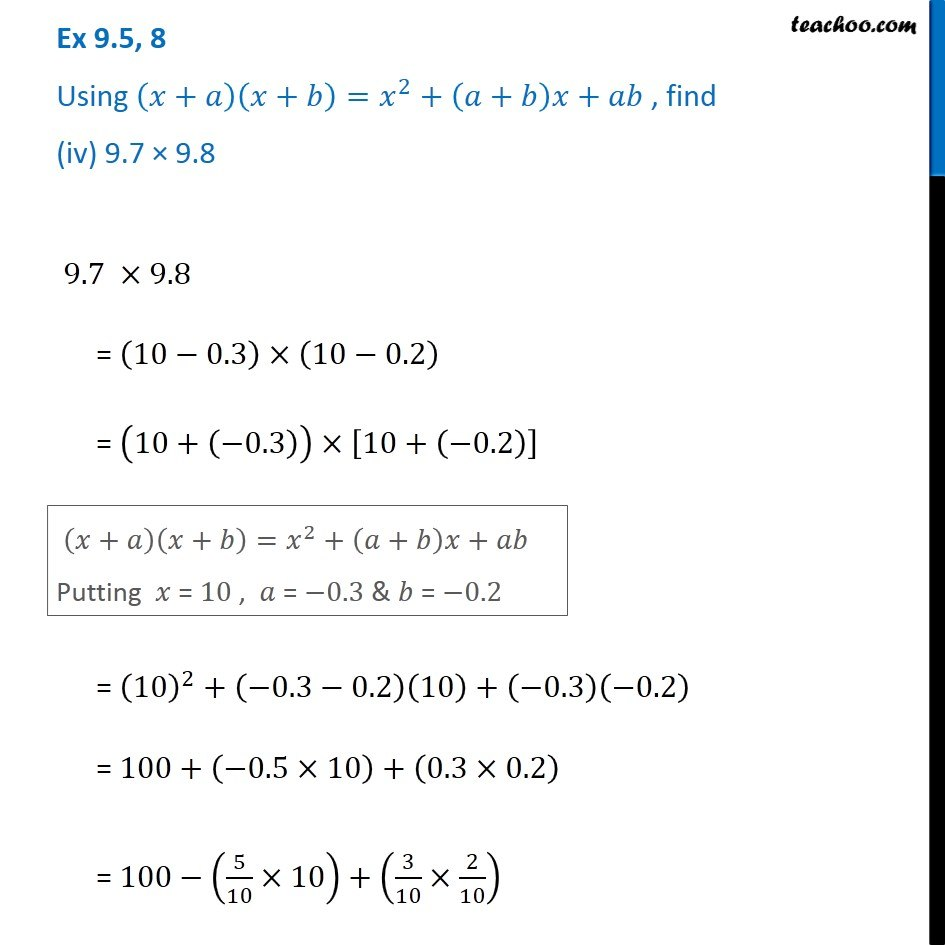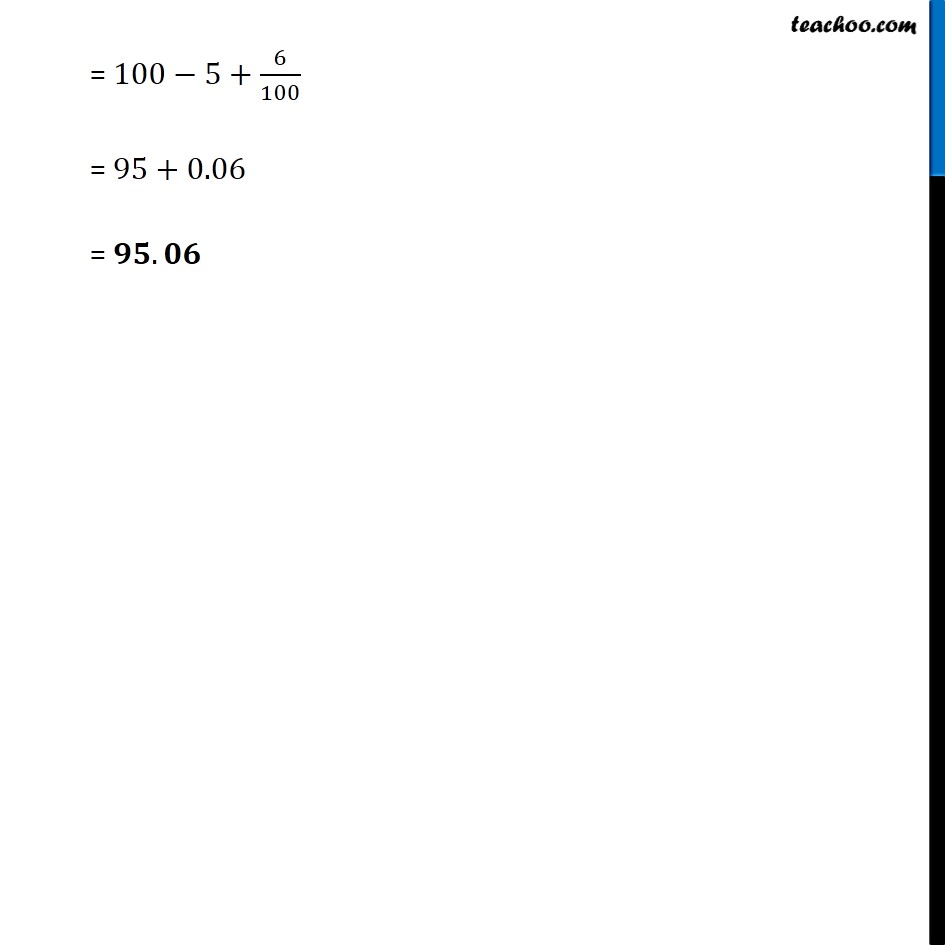1. Chapter 9 Class 8 Algebraic Expressions and Identities
2. Serial order wise
3. Ex 9.5

Transcript

Ex 9.5, 8 Using (𝑥+𝑎)(𝑥+𝑏)=𝑥^2+(𝑎+𝑏)𝑥+𝑎𝑏 , find (i) 103 × 104 103 × 104 = (100+3) ×(100+4) (𝑥+𝑎)(𝑥+𝑏)=𝑥^2+(𝑎+𝑏)𝑥+𝑎𝑏 Putting 𝑥 = 100 , 𝑎 = 3 & 𝑏 = 4 (𝑥+𝑎)(𝑥+𝑏)=𝑥^2+(𝑎+𝑏)𝑥+𝑎𝑏 Putting 𝑥 = 100 , 𝑎 = 3 & 𝑏 = 4 Ex 9.5, 8 Using (𝑥+𝑎)(𝑥+𝑏)=𝑥^2+(𝑎+𝑏)𝑥+𝑎𝑏 , find (ii) 5.1 × 5.2 5.1 × 5.2 = (5+0.1)×(5+0.2) (𝑥+𝑎)(𝑥+𝑏)=𝑥^2+(𝑎+𝑏)𝑥+𝑎𝑏 Putting 𝑥 = 5 , 𝑎 = 0.1 & 𝑏 = 0.2 = (5)^2+(0.1+0.2)(5)+(0.1)(0.2) = 25+(0.3)5+(0.1×0.2) = 25+3/10×5+(1/10×2/10) = 25+(15/10)+2/100 = 25+1.5+0.02 = 𝟐𝟔.𝟓𝟐 Ex 9.5, 8 Using (𝑥+𝑎)(𝑥+𝑏)=𝑥^2+(𝑎+𝑏)𝑥+𝑎𝑏 , find (iii) 103 × 98 103 ×98 = (100+3)×(100−2) = (100+3)×[100+(−2)] (𝑥+𝑎)(𝑥+𝑏)=𝑥^2+(𝑎+𝑏)𝑥+𝑎𝑏 Putting 𝑥 = 100 , 𝑎 = 3 & 𝑏 = −2 = (100)^2+(3+2)(100)+(3)(−2) = 10000+100−6 = 10000+94 = 𝟏𝟎𝟎𝟗𝟒 Ex 9.5, 8 Using (𝑥+𝑎)(𝑥+𝑏)=𝑥^2+(𝑎+𝑏)𝑥+𝑎𝑏 , find (iv) 9.7 × 9.8 9.7 ×9.8 = (10−0.3)×(10−0.2) = (10+(−0.3))×[10+(−0.2)] (𝑥+𝑎)(𝑥+𝑏)=𝑥^2+(𝑎+𝑏)𝑥+𝑎𝑏 Putting 𝑥 = 10 , 𝑎 = −0.3 & 𝑏 = −0.2 = (10)^2+(−0.3−0.2)(10)+(−0.3)(−0.2) = 100+(−0.5×10)+(0.3×0.2) = 100−(5/10×10)+(3/10×2/10) = 100−5+6/100 = 95+0.06 = 𝟗𝟓.𝟎𝟔

Ex 9.5